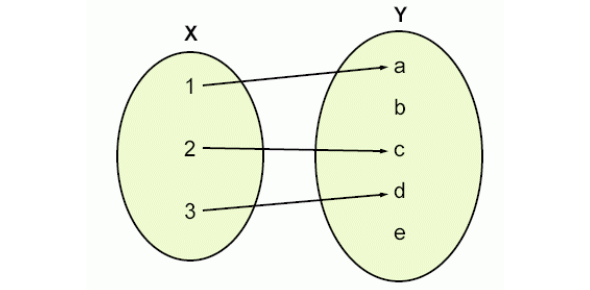# Sets And Functions Multiple Choice Questions & Answers

10 Questions | Attempts: 87SettingsIt's time to unleash your mathematical genius? Take our online quiz and solve questions about sets and functions to test yourself and prepare for an upcoming exam.

• 1.
Let f : X → X such that f(f (x )= x for all x∈ X then
• A.

F is one- to- one and onto

• B.

F is one- to- one but not onto

• C.

F is onto but not one-to -one

• D.

F need not be either one- to -one or onto

• 2.
Let A be a closed subset of RA≠∅  and   A≠R . Then A is
• A.

The closure of the interior of A

• B.

A countable set

• C.

A compact set

• D.

Not open

• 3.
Which of the following is/are true?
• A.

(1+ 1 /n) n +1 → e   as n →∞

• B.

(1+ 1 /n+1 )n →e  as n→∞

• C.

(1+ 1 /n )n 2 →e  as n→∞

• D.

(1+ 1 /n 2) n →e  as n→∞

• 4.
Let X⊂R  be an infinite countable bounded subset  of R  which of the statements is true
• A.

X cannot be compact

• B.

X contains an interior point

• C.

X may be closed

• D.

Closure of X is countable

• 5.
Which is compact in R n ?
• A.

{x1,x2,x3,……….xn   : xi<1,   1≤i≤n}

• B.

{x 1 , x 2 , x 3 ,………. x n   : x 1 + x 2 + x 3 ……. x n =0}

• C.

{x 1 , x 2 , x 3 ,………. x n : x i ≥0,  1≤i≤n}

• D.

{x 1 , x 2 , x 3 ,………. x n :   1≤x i ≤2,  1≤i≤n }

• 6.
Let I={1}∪{2} for x∈R let ϕ (x) =dist {x,I} =Inf{ |x-y |:y∈I} then is
• A.

Discontinuous somewhere

• B.

Continuous on R but differentiable only at x=1

• C.

Continuous on R but differentiable only at x=1,2

• D.

Continuous on R but not differentiable only at   x=1, 3/ 2 ,2

• 7.
Suppose f : R→R  is a function that satisfies  |f(x) -f(y)| ≤ |x-y| β, β>0 then
• A.

If β=1 then f is differentiable

• B.

If β>0 then f is uniform continuous

• C.

If β>1  then f is constant function

• D.

F must be a polynomial

• 8.
Which of the following subsets of  R2  is /are convex
• A.

{(x,y):  |x|≤5 , |y|≤10}

• B.

{(x,y) :   x 2 + y 2 =1}

• C.

{(x,y) :  y ≥ x 2 }

• D.

{(x,y) :  y≤ x 2 }

• 9.
Consider the set X={(-∞,0)∪ 1/n, n ∈ N}⊂R  with the subspace topology. Then
• A.

0 is an isolated point.

• B.

(–2, 0] is an open set

• C.

0 is a limit point of the subset {1 /n ,n∈N}

• D.

(–2, 0) is an open set

• 10.
Let G 1 and G 2 be two subsets of   R 2 and  f: R 2 →R 2 be a function, then
• A.

F -1 (G 1 ∪ G 2 )= f -1 ( G 1 )∪ f -1 ( G 2 )

• B.

F -1 ( G 1) c = (f -1 ( G 1 )) c

• C.

F (G 1 ∩ G 2) =f (G 1 ) ∩ f ( G 2 )

• D.

If G1 is open and G2 is closed then G1+ G2 = {x+y : x∈ G1,y∈ G2 is neither open nor closed

## Related TopicsBack to top
×

Wait!
Here's an interesting quiz for you.